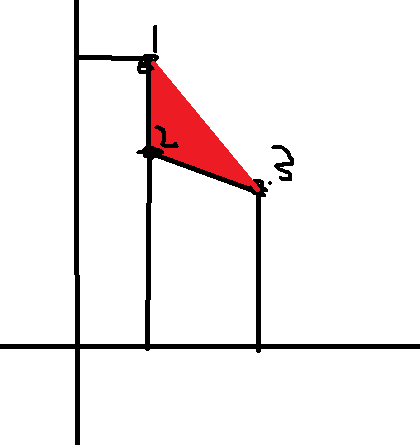{{ message }}

# A problem when calculating mAP#110

Closed
opened this issue Feb 25, 2019 · 10 comments
Closed

# A problem when calculating mAP#110

opened this issue Feb 25, 2019 · 10 comments

###budui commented Feb 25, 2019

Lines 47 to 54 in a253943

 for i in range(ngood): d_recall = 1.0/ngood precision = (i+1)*1.0/(rows_good[i]+1) if rows_good[i]!=0: old_precision = i*1.0/rows_good[i] else: old_precision=1.0 ap = ap + d_recall*(old_precision + precision)/2

In the above code, I can't understand the way to calculate `old_precision`. Here `old_precision` should be the precison of the last true-match, should it be changed like this?
`old_precision = i*1.0/(rows_good[i-1] +1)`
or just

```...
ap = ap + d_recall*(old_precision + precision)/2
# Simplified from here `http://www.robots.ox.ac.uk/~vgg/data/oxbuildings/compute_ap.cpp`
old_precision = precision```
We are unable to convert the task to an issue at this time. Please try again.

###budui commented Feb 25, 2019 • edited

 simple test: ```import numpy as np score = [0.6, 0.8, 0.1, 0.9, 0.2] index = np.argsort(score)[::-1] good_index = [3, 0] ngood = len(good_index) mask = np.in1d(index, good_index) rows_good = np.argwhere(mask==True) rows_good = rows_good.flatten() print("index:", index) print("good_index:", good_index) print("\nrows_good:", rows_good)``` ``````index: [3 1 0 4 2] good_index: [3, 0] rows_good: [0 2] `````` origin way ```ap = 0 for i in range(ngood): d_recall = 1.0/ngood precision = (i+1)*1.0/(rows_good[i]+1) if rows_good[i]!=0: old_precision = i*1.0/rows_good[i] else: old_precision=1.0 print("{0} (old_precision, precision) = ({1}, {2})".format(i, old_precision, precision)) ap = ap + d_recall*(old_precision + precision)/2 print("ap:", ap)``` ``````0 (old_precision, precision) = (1.0, 1.0) 1 (old_precision, precision) = (0.5, 0.6666666666666666) ap: 0.7916666666666666 `````` way1 ```ap = 0 for i in range(ngood): d_recall = 1.0/ngood precision = (i+1)*1.0/(rows_good[i]+1) if rows_good[i]!=0: old_precision = i*1.0/(rows_good[i-1]+1) else: old_precision=1.0 print("{0} (old_precision, precision) = ({1}, {2})".format(i, old_precision, precision)) ap = ap + d_recall*(old_precision + precision)/2 print("ap:", ap)``` ``````0 (old_precision, precision) = (1.0, 1.0) 1 (old_precision, precision) = (1.0, 0.6666666666666666) ap: 0.9166666666666666 `````` way2 ```ap = 0 for i in range(ngood): d_recall = 1.0/ngood if i == 0: precision, old_precision = (i+1)*1.0/(rows_good[i]+1), (i+1)*1.0/(rows_good[i]+1) else: precision, old_precision = (i+1)*1.0/(rows_good[i]+1), precision print("{0} (old_precision, precision) = ({1}, {2})".format(i, old_precision, precision)) ap = ap + d_recall*(old_precision + precision)/2 print("ap:", ap)``` ``````0 (old_precision, precision) = (1.0, 1.0) 1 (old_precision, precision) = (1.0, 0.6666666666666666) ap: 0.9166666666666666 ``````

###layumi commented Feb 25, 2019 • edited

 If you want to use exactly same code with the original code, (in http://www.robots.ox.ac.uk/~vgg/data/oxbuildings/compute_ap.cpp ) you should not use the modified `for` loop. Note that I use `for i in range(ngood)`. You may use `for i in range(ngallery)`. `ngallery` is the number of all gallery images.

###budui commented Feb 25, 2019

 as you mentioned in #85 (comment) the value of mAP only changed when it meets the true-matches. In fact, I just want to correct your code, not using exactly same code with the original code. `old_precision` should be `i*1.0/(rows_good[i-1] +1)` rather than `i*1.0/rows_good[i]`, this is my point.

###layumi commented Feb 25, 2019

 if `i=0` ?

###budui commented Feb 25, 2019

 Sorry, I missed some boundary conditions. We use the example above ``````index [3 1 0 4 2] good_index: [3, 0] rows_good: [0 2] `````` the origin way get this ``````0 (old_precision, precision) = (1.0, 1.0) 1 (old_precision, precision) = (0.5, 0.6666666666666666) ap: 0.7916666666666666 `````` when `i=1` , origin code get wrong `old_precision` 0.5. but when `i=0`, `precision`=1, 0.5 is a wrong number. if the number of query and gallery are large enough, origin code's result is similar to the correct result.

###layumi commented Feb 25, 2019 • edited

 The original cpp code contains a long `for` to visit every elements in the ranking list. So they can use `old_precision = precision`. Note that precision is changing in every iteration!!! My python code only uses `for` to visit the right matches. `precision` hasn't been updated to the new value (for the next use) after every iteration. I use more direct way to assign the value for the real `old_precision`. `old_precision = i*1.0/rows_good[i]` It is wrong to use `old_precision = precision`.

###budui commented Feb 25, 2019

I'm confused:

I use more direct way to assign the value for the real `old_precision`.
`old_precision = i*1.0/(rows_good[i-1]+1)`

but

Line 51 in a253943

 old_precision = i*1.0/rows_good[i]

###budui commented Feb 25, 2019

 sorry for aggressive. I'm very confused now. I have test the example above with Market1501's MATLAB code. the result of it is the same as your code. Maybe I need more exercise。我现在觉得我很菜。 我刚写了篇关于mAP的博客，尝试写出mAP的公式，看了公式还是不知道为什么old_precision 要赋值为`i*1.0/rows_good[i]`。您能帮忙解答一下吗...

###budui commented Feb 25, 2019

 我搞懂了，不是上一个匹配点，而是上一个rank对应的点。谢谢。

###budui commented Feb 25, 2019

 I make a simple picture:I thought it should be average of point 1 and point 3, in fact we should use point 2 and point 3. Thanks for your patience @layumi.